Thursday , March 30 2023
Breaking News# Longevity Research for a New Theory of Pam Block

INTRODUCTION  BY BASILIOLI SANDRO  ( VEDIKO )

3 STEP PAM VEDIC THEORY ( PART 2 )

In my first article of my theory , I have showed the 1st step to find pam-1 using the three division charts of Drekkana, Navamsha and Dwadasamsha. The pointers I use after tying different ways are, Lagna and Hora Lagna. Here I am presenting the 1st table .

Note: I use Lahiri Ayamansa                                                                                                                          TABLE A

Short life                           Middle life                           Long life

S-S-S = 12y to 32y     S-M-M = 33y to 67y     S-L-L = 62y to 93y          M-S-S = 12y to 36y    M-M-M= 36y to 72y  M-L-L = 69y to 100y        L-S-S = 12y to 42y     L-M-M = 40y to 82y     L-L-L = 78 to108y          L-M-Ss = 12y to 44y  L-S-M = 34y to 70y      S-M-L = 60y to 91y

2nd EXTRA PAM BLOCK

In this 2nd Pam block we will use the Rasi chart to find the Extra Pam to try to reduce a little bit more the Longevity block. What we will do is , first get the longitude of the Lagna and sum with the longitude of Hora Lagna then moltliply  with the Pam ( for example : short life x 32, middle life x32 and long life x64 ) , then moltilply by 12 to get the days then divid  by 365 to get the years for reduction.  The next step is, lets say it is long life formula = L-L-L: 78y to 108y  in this case the reduction is from 120y and 100y to get the extra pam. Here I am presenting a table for reductions.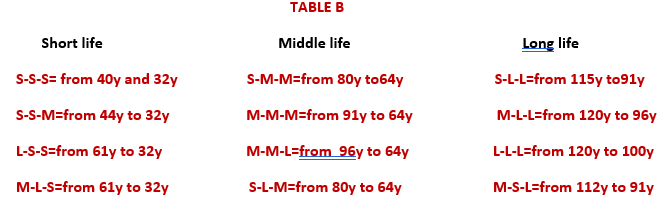The normal formula is: Longitude Lagna + Longitude HL /2 x Pam x 12 / 365 =  years for reduction. This formula can change depending on the formula of longevity ,here I am presenting a 3rd table that shows if the total longitude of Lagna and HL should be divided by 2 ,  by 4 or not at all.

TABLE  C

Short life cases

In all the cases : S-S-S , S-S-M , S-S-L, L-M-S , if total longitude Lagna + HL  is not more than 16°59 degree  no division is done, if more than 16°59 degree lets say 17°degree divide by 2.If after 2nd division the total is still more than 17°, divide again by 2.

Middle life cases

S-l-m and  S-M-M: total less than 26°59 degree,  if not divide by 2 . M-M-M and M-M-L: if total is up to 29°59 degree  no division has to be done, if  is 30° degree divide by 2 .

Long life cases

In long life M-L-L & L-L-L cases, if the total is more than 12°.59 degree division is done , If total is not more than 12°.59  no division is done . One more thing is ,if after the first division by 2 the total is more than 12°.59 degree divide again by 2 if after the 2nd division the total is still more than 12°.59 degree leave it as it is.  In the cases of codes S-M-L and S-L-L , if the total is up to 15°.59, no division is done . If after 1st division is still more than 15°59 ,divide again by 2.

It’s looks difficult but it is not so I will illustrate so one can understand the method.

ILLUSTRATIONS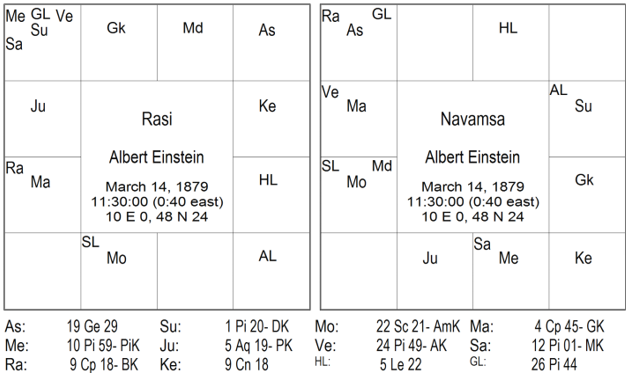Albert Einstein lived up to 76 years in the division chart D3 Lagna in libra and HL in leo a mutual and a fixed signs gives middle life = M, in D9 Lagna in pisces  and HL in taurus a dual and a fixed signs give long life= L , in D12 Lagna in Capricorn and HL in libra both mutual signs gives long life= L , so the formula is: M-L-L and looking at TABLE A is, from 69y to 100y. Now we want to go a little more closer to reduce the Pam with no dasha system so we have to find the Extra Pam, to get the Extra Pam we look at the Rasi chart and add the longitude of Lagna and HL. Long Lagna 19°29’ + long HL  5°22’ = 24,51 . 24°degree is more than 12°degree  TABLE C so we divide by 2 = 12.255 it’s not more than 16° table c, so you don’t divide again by 2. Then 12.255 x 64 ( because the life is long) = 78432  x 12 = 941184 (days) divide by 365 = 25.78(years for reduction). Now 25.78 becomes 26 years and in the TABLE B the reduction is from 120y to 96y for the M-L-L formula. 120-26= 94y    96-26= 70y  so Extra Pam is from 70y to 94y. It has reduced the Pam only a little but sometimes the difference is much more depend on the case.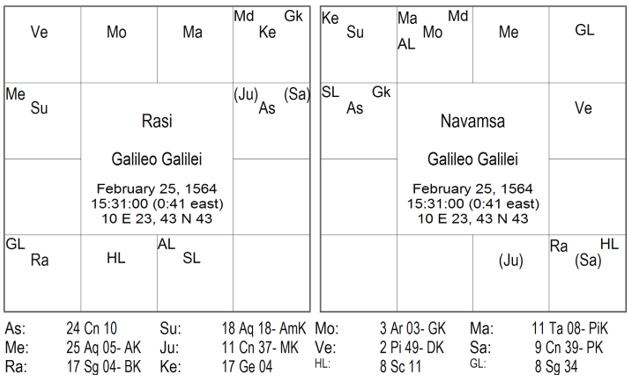Galileo Galilei lived upto 78years .In the D3 chart Lagna is in pisces  and HL in scorpion a dual and fixed signs it gives long life = L , in the D9 chart Lagna in acquarius and HL in virgo  signs a fixed and dual sign it gives a long life = L , in the D12 chart lagna is in aries  and hl in acquarius a mutual and fixed signs it gives a middle life = M , so the formula is: L-L-M and looking at TABLE A is, from 69y to100y now we want to reduce this difference to find the Extra Pam. In rasi chart longitude of Lagna is 24°10 ‘+ longitude HL 8°11’=32.21 ,it is more than 12°degree  TABLE C so we divide by 2 =16.105 it is more than 12°degree so we divide again by 2 =8.0525 multliply by the Pam it is long life so by 64y, 08.0525×64=51536(days) x 12=618432(months) divided by 365=16.94(years for reduction) = 17 years. In TABLE B we look for the right reductions for L-L-M formula . 120-17= 103      96-17= 79y so Extra Pam is: from79y to 103y. difference from first cycle is: 7 years reduced.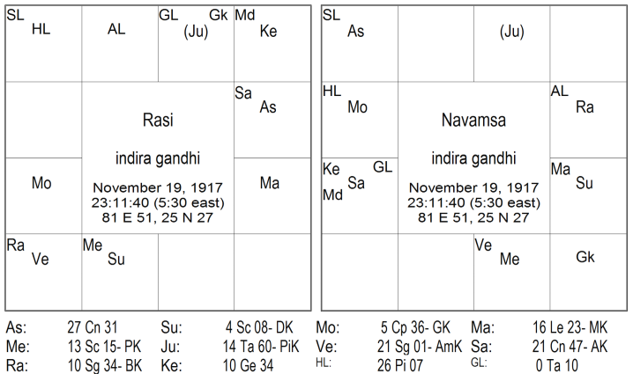Indira Gandhi lived upto 66years. In the division chart D3 Lagna is in pisces and HL in scorpion a dual and a fixed signs so the life is long= L , in D9 Lagna in in pisces HL in scorpion a dual and a fixed signs so the life is long= L in D12 Lagna in twins HL in Capricorn a dual and a mutual signs so the life is short= S ,so the formula is: L-L-S  and looking at TABLE A is, from 62y to 93y now we want to reduce the difference so find the Extra Pam. In Rasi chart longitude Lagna is : 27°31’ + HL is 26°07’ =53.37 / 2 =26.685 /2=13.34 . 13.34 is more than 12°deg after the 2nd division, it means the lifespan wont be so long so leave it and go a head TABLE C. 13.34×64=85376×12=1024512/365= 28.06y = 29year for reductions. In TABLE B the formula S-L-L reductions is from 115 to 91y. 115-29=87y    91-29=63y . The Extra Pam is from 63y to 87y. Difference from first cycle is, 10 years reduced .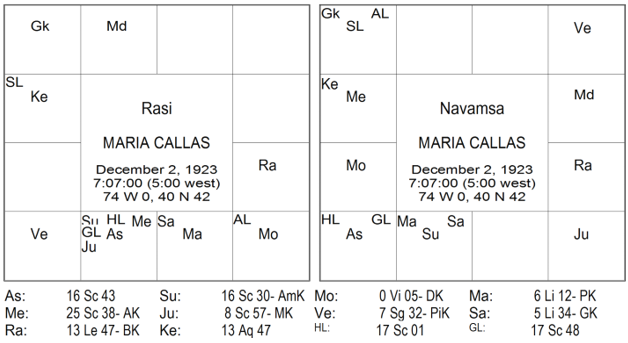Maria Callas lived upto 53years . In the D3 chart Lagna and HL in pisces a dual sign so the life is middle = M  in D9 Lagna and HL in Sagittarius  a dual sign again is middle life= M  in D12 Lagna and HL in torius sign and life is short= S , so the formula is M-M-S  and looking at TABLE A goes from 33y to 67y. In Rasi chart longitude lagna :16°43’ + hl 17°01’=33.44 /2=16.72×32=53504×12=642048/365=17.59 = 17.59 becomes 18years for reduction.

In TABLE B  M-M-S  formula  reductions goes from 80y to 64y.

80-18=62y           64-18=46y so the Extra Pam is from 46 to 62 years. The difference from 1st cycle is 18years reduced.

In the next article I will give a thesis of all the theory, giving the same horoscopes so you can understand how to proceed .